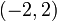# Special linear group:SL(2,R)

View a complete list of particular groups (this is a very huge list!)[SHOW MORE]

## Definition

The group$SL(2,\R)$ is defined as the group of$2 \times 2$ matrices with entries from the field of real numbers and determinant$1$, under matrix multiplication.$SL(2,\R) := \left \{ \begin{pmatrix} a & b \\ c & d \\\end{pmatrix} \mid a,b,c,d \in \R, ad - bc = 1 \right \}$.

It is a particular case of a special linear group over reals and hence of a special linear group.

### Structures

The group has the structure of a topological group, a real Lie group, and an algebraic group restricted to the reals.

## Arithmetic functions

Function Value Similar groups Explanation
order of a group cardinality of the continuum The cardinality is at least that of the continuum, because we can inject$\R$ into this group by$x \mapsto \begin{pmatrix} 1 & x \\ 0 & 1 \\\end{pmatrix}$. On the other hand, it is a subset of$\R^4$, so the cardinality is not more than that of the continuum.
exponent of a group infinite there exist elements, such as$\begin{pmatrix} 1 & 1 \\ 0 & 1 \\\end{pmatrix}$, of infinite order.
composition length 2 groups with same composition length Center is simple (isomorphic to cyclic group:Z2) and the quotient group PSL(2,R) is also simple.
chief length 2 groups with same chief length Similar reason to composition length.
dimension of an algebraic group 3 groups with same dimension of an algebraic group As$SL(n,\_), n = 2: n^2 - 1 = 2^2 - 1 = 3$
dimension of a real Lie group 3 groups with same dimension of a real Lie group As$SL(n,\R), n = 2: n^2 - 1 = 2^2 - 1 = 3$

## Group properties

### Abstract group properties

Property Satisfied? Explanation
abelian group No
nilpotent group No
solvable group No
quasisimple group Yes special linear group is quasisimple (with a couple of finite exceptions). Its inner automorphism group, which is projective special linear group:PSL(2,R), is simple.
simple non-abelian group No The center is$\pm I$, so is proper and nontrivial.

### Topological/Lie group properties

Property Satisfied? Explanation
connected topological group Yes It is generated by matrices of the form$\begin{pmatrix} 1 & x \\ 0 & 1 \\\end{pmatrix}, x \in \R$ and$\begin{pmatrix} 1 & 0 \\ x & 1 \\\end{pmatrix}, x \in \R$. Both sets are connected sets are matrices containing the identity, so the group is connected.
compact group No It contains matrices of the form$\begin{pmatrix} 1 & x \\ 0 & 1 \\\end{pmatrix}, x \in \R$ where the$x$ can be arbitrarily large, so is not compact as a subset of$\R^4$.
simply connected group No The fundamental group is isomorphic to the group of integers. The group has$n$-fold coverings for every natural number$n$.
semisimple Lie group Yes
semisimple algebraic group Yes
reductive algebraic group Yes

## Elements

Further information: element structure of special linear group:SL(2,R)

Below is a summary of the conjugacy class structure:

Nature of conjugacy class Eigenvalues Characteristic polynomial Minimal polynomial What set can each conjugacy class be identified with? (rough measure of size of conjugacy class) What can the set of conjuacy classes be identified with (rough measure of number of conjugacy classes) What can the union of conjugacy classes be identified with? Semisimple? Diagonalizable over$\R$? Splits in$SL_2$ relative to$GL_2$?
Diagonalizable over$\R$ with equal diagonal entries, hence a scalar$\{ 1,1 \}$ or$\{ -1,-1\}$$(x - a)^2$ where$a \in \{ -1,1 \}$$x - a$ where$a \in \{ -1,1\}$ one-point set two-point set two-point set Yes Yes No
Parabolic conjugacy class: Not diagonal, has Jordan block of size two$1$ (multiplicity 2) or$-1$ (multiplicity 2)$(x - a)^2$ where$a \in \{ -1,1 \}$ Same as characteristic polynomial  ? four-point set, two for eigenvalue 1, two for eigenvalue -1  ? No No Both the$GL_2$-conjugacy classes split into two pieces.
Elliptic conjugacy class: Diagonalizable over$\mathbb{C}$ but not over$\R$. Must necessarily have no repeated eigenvalues. Pair of conjugate elements in$\mathbb{C}$ of modulus 1$x^2 - ax + 1$,$-2 \le a \le 2$ Same as characteristic polynomial  ? direct product of the open interval$(-2,2)$ with a two-point set  ? Yes No each$GL_2$-conjugacy class splits into two$SL_2$-conjugacy classes.
Hyperbolic conjugacy class: Diagonalizable over$K$ with distinct (and hence mutually inverse) diagonal entries$\lambda, 1/\lambda$ where$\lambda \in \R \setminus \{ 0,1,-1 \}$$x^2 - (\lambda + 1/\lambda)x + 1$ Same as characteristic polynomial  ?$\R \setminus [-2,2]$  ? Yes Yes No
Total NA NA NA NA  ?  ?  ?  ?  ?

## Supergroups

Further information: supergroups of special linear group:SL(2,R)

### Quotients: Schur covering groups

Further information: group cohomology of special linear group:SL(2,R)

The fundamental group of$SL(2,\R)$, viewed as a topological group, is the group of integers. This is therefore also the second cohomology group. The upshot is that the Schur covers of this group are the same as the topological covers, and these correspond to all the possible quotient groups of the fundamental group.

The universal covering group is a group with central subgroup$\mathbb{Z}$ and quotient SL(2,R) (see universal covering group of SL(2,R)). In addition, for every positive integer$n$, there is a$n$-fold cover with central subgroup cyclic of order$n$ having quotient$SL(2,\R)$ (the actual center is cyclic of order$2n$ and the inner automorphism group is PSL(2,R)).

Of particular interest is the case$n = 2$. The 2-fold cover of$SL(2,\R)$ is metaplectic group:Mp(2,R). This has center isomorphic to cyclic group:Z4 and inner autmoorphism group isomorphic to PSL(2,R).

## Subgroups

Further information: subgroup structure of special linear group:SL(2,R)